# Calculus 1 : How to find local maximum graphing functions of curves

## Example Questions

1 2 4 Next →

### Example Question #31 : Curves

Find all the x values which have a local maxima of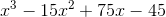.

Graph to confirm your answer.

Possible Answers: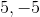None of the other answers.

Correct answer:

None of the other answers.

Explanation:

We need to differentiate term by term, applying the power rule,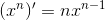This gives us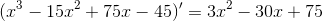The critical points are the points where the derivative equals 0. To find those, we can use the quadratic formula: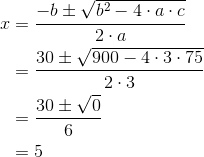Any local maximum will fall at a critical point where the derivative passes from positive to negative. To check this, we check a point in each of the intervals defined by the critical points: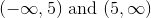.

Let's take 0 from the first interval, and 6 from the second interval.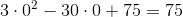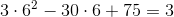The derivative stays positive on either side of the critical point, so this function doesn't have a maximum.

### Example Question #32 : Curves

Find the x value for the local maxima of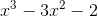.

Possible Answers:None of the other answers.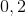Correct answer:Explanation:

We need to differentiate term by term, applying the power rule,This gives us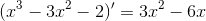The critical points are the points where the derivative equals 0. To find those, we can use the quadratic formula: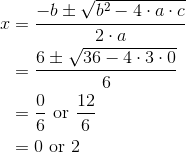Any local maximum will fall at a critical point where the derivative passes from positive to negative. To check this, we check a point in each of the intervals defined by the critical points: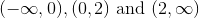.

Let's take -1 from the first interval, 1 from the second interval, and 3 from the third interval.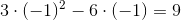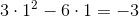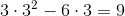The derivative moves from positive to negative at 0, so that is the function's only local maximum.

### Example Question #33 : Curves

Determine the local maxima of the following function: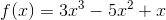Possible Answers: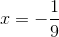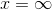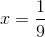Correct answer:Explanation:

To find the local maxima of the function, we must determine the x values at which the first derivative of the function changes from positive to negative.

The first derivative is equal to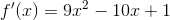and was found using the following rule: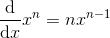Next, we must find the critical values, or the values at which the first derivative of the function is equal to zero: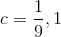Using the critical values, we can create our intervals on which we examine the sign of the first derivative: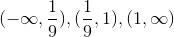Note that at the endpoints of the intervals, the first derivative is neither positive nor negative.

Next, check the sign of the first derivative on each interval by plugging in any value on the interval into the first derivative function and checking the sign. On the first interval, the first derivative is positive, on the second, it is negative, and on the third, it is positive. Thus, a local maximum exists at the value at which the first derivative changed from positive to negative,.

### Example Question #34 : Curves

Determine the local maxima for the following function: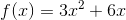Possible Answers:There are no local maxima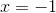Correct answer:

There are no local maxima

Explanation:

To determine the local maxima of the function, we must find the points at which the first derivative of the function changes from positive to negative.

The first derivative of the function is equal to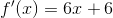and was found using the following rule:Next, we find the critical values, at which the first derivative is equal to zero: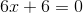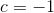Using the critical value, we can make the intervals on which we check the sign of the first derivative: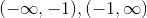To check the sign of the first derivative on each interval, simply plug in any point on each interval into the first derivative and check the sign. On the first interval, it is negative, but on the second it is positive. The first derivative never changes from positive to negative, so the function has no local maxima.

### Example Question #35 : Curves

Determine the local maxima for the following function: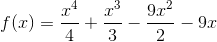Possible Answers: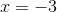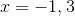Correct answer:Explanation:

To determine the local maxima for the function, we must determine where the first derivative changes from positive to negative.

First, we must find the first derivative: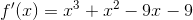The derivative was found using the following rule:Next, we must find the critical values, at which the first derivative is equal to zero: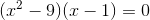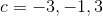Using the critical values, we can make the intervals on which we check the sign of the first derivative: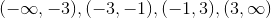Note that at the endpoints of each interval, the first derivative is neither positive nor negative.

To check the sign of the first derivative, simply plug in any value on each interval into the first derivative function and check the sign. On the first interval, the derivative is negative, on the second it is positive, on the third it is negative, and on the fourth it is positive. Between the second and third intervals is the change indicating a local maximum, at.

### Example Question #36 : Curves

Determine the local maxima for the following function: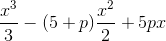where p is a constant,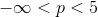.

Possible Answers: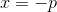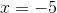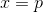Correct answer:Explanation:

To determine the local maxima for the following function, we must determine where the first derivative of the function changes from positive to negative.

The first derivative of the function is equal to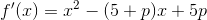and was found using the following rule:Now, we must find the critical values, at which the first derivative is equal to zero: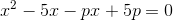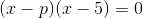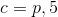Using the critical values, we can make the intervals on which we check the sign of the first derivative: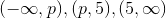Note that because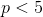, it comes before 5 when making the intervals. Also, note that at the endpoints of the intervals the first derivative is neither positive nor negative.

Now, we can check the sign of the first derivative by plugging in any value on each interval into the first derivative. It may seem daunting havinginstead of a number, but to simplify your thinking, you can let the variable be any number less than 5. After doing this, and plugging in numbers on each interval, we find that on the first interval, the first derivative is positive, on the second it is negative, and on the third it is positive. A sign change from positive to negative occured at, so the local maximum exists here.

1 2 4 Next →

### All Calculus 1 Resources